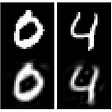# Some Context

During the month of November, I had the opportunity to be a part of a consulting challenge for Instacart! The challenge was to come up with a solution to improve customer service at Instacart. My group decided to target the produce issue at Instacart since it has been known that some consumers have received rotten produce from shoppers.

To put things simply, my group’s solution was to create live produce detection that would detect if the produce was rotten (mould, discoloured) or fresh (meaning it was good enough quality to buy) and then verify based on the results if the shopper was allowed to purchase the product.

During this challenge, I had the opportunity to create my own deep learning model to detect rotten vs fresh fruits as a prototype for our solution.

The process was definitely stressful because this was the 2nd deep learning project I had ever done. I had to build 6 different models (3 in PyTorch and 3 in TensorFlow), and I failed a lot. I’m glad I persisted tho because my group was able to send in the prototype to Instacart!

During this article, I’m going to talk about image detection in the fruit industry and how I built my model, so stay tuned :)

# Importance

Using A.I. to detect food is a growing industry. With the power of machine learning, we can detect unripe, contaminated, and rotten fruit.

Companies such as ImpactVision leverage machine learning alongside hyperspectral imaging, which essentially combines spectroscopy and computer vision. Doing so allows computers to access different properties of food such as quality.

Using this combination of technologies can allow companies to actually reduce their waste, and eventually, consumers will be able to go shopping and scan their phones on an avocado to determine if it’s ripe enough. Not only does hyperspectral imaging and machine learning greatly impact the food industry, but this combination is also being put forward in the medical industry to work on detecting cancer.

Although my prototype does not make use of hyperspectral imaging, I focused on the computer vision aspect of it.

# The Neural Network

I built this CNN in both PyTorch and Keras Tensorflow, and I will include examples from both libraries. In this overview, I’m assuming you understand intermediate python concepts (object-oriented programming, loops, functions, different data types, external libraries).

If you need a refresher on how CNNs work, check out this article I wrote explaining them:

# Dataset Information

This dataset contains:

• images of rotten apples
• images of rotten bananas
• images of rotten oranges
• images of fresh apples
• images of fresh bananas
• images of fresh oranges
• There are 5501 training images
• There are 1384 testing images

Down below is where I got the data for this project:

# Overview Of The Network

Here is an overview of the code we will be working with.

## Tensorflow

`#importsimport tensorflow as tffrom tensorflow.keras.models import Sequentialfrom tensorflow.keras.layers import Dense, Flatten, Dropout, Conv2D, MaxPooling2Dfrom tensorflow.keras.preprocessing.image import ImageDataGenerator#loading directories + data manipulationtrain_path = '/Users/ashleyc/Deeplearning/fresh_and_rotton/dataset/train'test_path = '/Users/ashleyc/Deeplearning/fresh_and_rotton/dataset/test'BATCH_SIZE = 10train_batches = ImageDataGenerator(    preprocessing_function=tf.keras.applications.vgg16.preprocess_input,    rescale=1/255.,    horizontal_flip=True,    vertical_flip=True).flow_from_directory(    directory=train_path,    target_size=(20, 20),    classes=['freshapples', 'freshbananas', 'freshoranges', 'rottenapples', 'rottenbananas','rottenorganges'],    batch_size=BATCH_SIZE,    class_mode='categorical',    color_mode='rgb')test_batches = ImageDataGenerator(    preprocessing_function=tf.keras.applications.vgg16.preprocess_input, rescale=1/255.).flow_from_directory(    directory=test_path,    target_size=(20, 20),    classes=['freshapples', 'freshbananas', 'freshoranges', 'rottenapples', 'rottenbananas','rottenorganges'],    batch_size=BATCH_SIZE,    class_mode='categorical',    color_mode='rgb',    shuffle=False)#building the modelmodel = Sequential()model.add(Conv2D(32, (3, 3), activation=('relu'), input_shape=(20, 20, 3)))model.add(MaxPooling2D((2, 2)))model.add(Conv2D(64,(3,3), activation=('relu')))model.add(MaxPooling2D(2,2))model.add(Flatten())model.add(Dense(128, activation=('relu')))model.add(Dense(128, activation=('relu')))model.add(Dense(6, activation=('softmax')))#evaluating the modelmodel.compile(optimizer='adam', loss='categorical_crossentropy', metrics=['accuracy'])model.fit(test_batches, epochs=17)`

## PyTorch

`#importsimport torchfrom torchvision import datasets, models, transforms, utilsimport torch.nn as nnimport torch.optim as optimimport osimport numpy as npimport matplotlib.pyplot as pltimport torch.nn.functional as F#loading directories + data manipulationfruit_train = '/Users/ashleyc/Deeplearning/fresh_and_rotton/dataset/train'fruit_test = '/Users/ashleyc/Deeplearning/fresh_and_rotton/dataset/test'data_dir = "/Users/ashleyc/Deeplearning/fresh_and_rotton/dataset"data_transform = {'train':transforms.Compose([                    transforms.Resize((224, 224)),                    transforms.RandomHorizontalFlip(),                    transforms.ToTensor(),                    transforms.Normalize([0.485, 0.456, 0.406], [0.229, 0.224, 0.225])                    ]),                  'test':transforms.Compose([                      transforms.Resize((224, 224)),                      transforms.ToTensor(),                      transforms.Normalize([0.485, 0.456, 0.406], [0.229, 0.224, 0.225])                  ]) }image_datasets = {x: datasets.ImageFolder(os.path.join(data_dir, x), data_transform[x]) for x in ['train', 'test']}data_loader = {x:torch.utils.data.DataLoader(image_datasets[x], shuffle=True, batch_size=124, num_workers=0) for x in ['train', 'test']}class_names = image_datasets['train'].classes#visualization of datadef imshow(inp, title=None):    inp = inp.numpy().transpose((1, 2, 0))    mean = np.array([0.485, 0.456, 0.406])    std = np.array([0.229, 0.224, 0.225])    inp = std * inp + mean    inp = np.clip(inp, 0, 1)    plt.imshow(inp)    if title is not None:        plt.title(title)    plt.pause(0.001)inputs, classes = next(iter(data_loader['train']))out = utils.make_grid(inputs)imshow(out, title=[class_names[x] for x in classes])#building the networkclass Net(nn.Module):    def __init__(self):        super().__init__()        self.conv1 = nn.Conv2d(3, 16, kernel_size=3, padding=1)        self.conv2 = nn.Conv2d(16, 8, kernel_size=3, padding=1)        self.fc1 = nn.Linear(8*56*56, 56) #256        self.fc2 = nn.Linear(56, 6)        self.relu = nn.ReLU()    def forward(self, x):        x = F.max_pool2d(self.relu(self.conv1(x)), 2)        x = F.max_pool2d(self.relu(self.conv2(x)), 2)        x = torch.flatten(x, 1)        x = self.relu(self.fc1(x))         x = self.fc2(x)        return xnet = Net()optimizer = optim.Adam(net.parameters(), lr=0.0001)cross_el = nn.CrossEntropyLoss()EPOCHS = 8#training the modelfor epoch in range(EPOCHS):    net.train()    for data in data_loader['train']:        x, y = data        net.zero_grad()        output = net(x)        loss = cross_el(output, y)        loss.backward()        optimizer.step()correct = 0total = 0#testing the modelwith torch.no_grad():    for data in data_loader['test']:        x, y = data        output = net(x)        for idx, i in enumerate(output):            if torch.argmax(i) == y[idx]:                correct +=1            total +=1print(f'accuracy: {round(correct/total, 3)}')`

This might look overwhelming, but don’t worry! I will break this down and explain the components.

Also please note, in the TensorFlow example, I used transfer learning but I did not use transfer learning for the PyTorch example. The TensorFlow model had an accuracy of 98 percent, as opposed to the PyTorch model which had a 92 percent accuracy, and the difference in accuracy could have been a result of me using transfer learning for the TensorFlow model.

# Imports

Let’s start off with the imports.

## TensorFlow

`import tensorflow as tffrom tensorflow.keras.models import Sequentialfrom tensorflow.keras.layers import Dense, Flatten, Conv2D,MaxPooling2Dfrom tensorflow.keras.preprocessing.image import ImageDataGenerator`

I firstly imported tensorflow as tf.

Then I imported the Sequential model from Keras.

I also imported Dense, Flatten, Conv2D, and MaxPooling2d, which we need for the layers of our model.

Lastly, I imported ImageDataGenerator from Keras’ preprocessing.image, and we need this for data augmentation.

## PyTorch

`import torchfrom torchvision import datasets, transforms, utilsimport torch.nn as nnimport torch.optim as optimimport osimport numpy as npimport matplotlib.pyplot as pltimport torch.nn.functional as F`

First, I imported torch.

Then I imported datasets, transforms, and utils for dataset manipulation and directory manipulation.

For my actual model structure, I imported torch.nn , torch.optim, and torch.nn.functional.

Additionally, I imported os, and in this case, the primary use of os was for manipulating directories.

For mathematical performances and operations, I imported NumPy.

# Directories and Data Manipulation

## TensorFlow

`train_path = '/Users/ashleyc/Deeplearning/fresh_and_rotton/dataset/train'test_path = '/Users/ashleyc/Deeplearning/fresh_and_rotton/dataset/test'BATCH_SIZE = 10train_batches = ImageDataGenerator(    preprocessing_function=tf.keras.applications.vgg16.preprocess_input,    rescale=1/255.,    horizontal_flip=True,    vertical_flip=True).flow_from_directory(    directory=train_path,    target_size=(20, 20),    classes=['freshapples', 'freshbananas', 'freshoranges', 'rottenapples', 'rottenbananas','rottenorganges'],    batch_size=BATCH_SIZE,    class_mode='categorical',    color_mode='rgb')test_batches = ImageDataGenerator(    preprocessing_function=tf.keras.applications.vgg16.preprocess_input, rescale=1/255.).flow_from_directory(    directory=test_path,    target_size=(20, 20),    classes=['freshapples', 'freshbananas', 'freshoranges', 'rottenapples', 'rottenbananas','rottenorganges'],    batch_size=BATCH_SIZE,    class_mode='categorical',    color_mode='rgb',    shuffle=False)`

Alright, now let’s break this big chunk of text down.

`train_path = '/Users/ashleyc/Deeplearning/fresh_and_rotton/dataset/train'test_path = '/Users/ashleyc/Deeplearning/fresh_and_rotton/dataset/test'`

The first step before we do any data manipulation, we need to initialize the directories.

`BATCH_SIZE = 10`

Our batch size refers to the number of items from the dataset our model is fed. I like to create variables for parameters that have numbers that might be changed within the model training purposes. This is because I only have to refer to a variable at the top of my code, rather than looking through my entire network to find this parameter.

`train_batches = ImageDataGenerator(    preprocessing_function=tf.keras.applications.vgg16.preprocess_input,    rescale=1/255.,    horizontal_flip=True,    vertical_flip=True).flow_from_directory(    directory=train_path,    target_size=(20, 20),    classes=['freshapples', 'freshbananas', 'freshoranges', 'rottenapples', 'rottenbananas','rottenorganges'],    batch_size=BATCH_SIZE,    class_mode='categorical',    color_mode='rgb')test_batches = ImageDataGenerator(    preprocessing_function=tf.keras.applications.vgg16.preprocess_input, rescale=1/255.).flow_from_directory(    directory=test_path,    target_size=(20, 20),    classes=['freshapples', 'freshbananas', 'freshoranges', 'rottenapples', 'rottenbananas','rottenorganges'],    batch_size=BATCH_SIZE,    class_mode='categorical',    color_mode='rgb',    shuffle=False)`

We have two ImageDataGenerators, one for our training batches and one for our testing batches. The purposes of these generators are to perform data augmentations, which will then be fed into our CNN.

The training and testing batches almost have identical generators with the exception of a few added features that the training batches have. We will examine the training batch generator and differentiate the added features.

`train_batches = ImageDataGenerator(    preprocessing_function=tf.keras.applications.vgg16.preprocess_input,    rescale=1/255.,    horizontal_flip=True,    vertical_flip=True).flow_from_directory(    directory=train_path,    target_size=(20, 20),    classes=['freshapples', 'freshbananas', 'freshoranges', 'rottenapples', 'rottenbananas','rottenorganges'],    batch_size=BATCH_SIZE,    class_mode='categorical',    color_mode='rgb')`

For our generator, we will use transfer learning. Essentially what transfer learning does is reuse a pre-trained model on a new problem, and the model will then exploit its knowledge from a prior task to improve generalization on a future task.

To define our transfer learning feature, we will use the preprocessing_function and use the vgg16 model.

`reprocessing_function=tf.keras.applications.vgg16.preprocess_input,`

Additionally, we need to rescale our image to 1/255., and this normalizes our inputs and transforms every pixel in the range 0,255 to 0,1. We do this to treat all the images in the same manner because different images have varying pixel ranges.

`rescale=1/255.,`

For our train_batches, we will apply a horizontal and vertical flip, but we will not do this for our testing data. When we feed in our testing data, we don’t want to augment it, we want to make sure the data is completely new in a sense. Keep in mind that for our test_batches, we need to set our shuffle to False.

`horizontal_flip=True,vertical_flip=True`

On our ImageDataGenerator, we will need to call the flow_from_directory function. This method will take in a directory path and generate batches of augmented data.

`.flow_from_directory(    directory=train_path,    target_size=(20, 20),    classes=['freshapples', 'freshbananas', 'freshoranges', 'rottenapples', 'rottenbananas','rottenorganges'],    batch_size=BATCH_SIZE,    class_mode='categorical',    color_mode='rgb')`

Within our flow_from_directory function, we will define our directory, which we initialized earlier as “train_path”.

`directory=train_path`

Then we need to reshape our images using the target_size parameter to (20,20).

`target_size=(20, 20)`

After we will define our classes, and in this model we have six.

`classes=['freshapples', 'freshbananas', 'freshoranges', 'rottenapples', 'rottenbananas','rottenorganges']`

Once you define your classes in TensorFlow, if you do this correctly, you’ll see this as an output in your terminal:

Found 5501 images belonging to 6 classes.
Found 1384 images belonging to 6 classes.

If you see this, you have defined your classes successfully.

Now we will define our batch_size using the BATCH_SIZE variable we initialized earlier.

`batch_size=BATCH_SIZE`

We will use categorical for our class mode because we have data that falls into one of many categories. In this case, we are classifying fruits, but there are six categories that the data can fall under. Additionally, we will want to define our color_mode as RGB since our images are RGB.

`class_mode='categorical',color_mode='rgb'`

## PyTorch

`fruit_train = '/Users/ashleyc/Deeplearning/fresh_and_rotton/dataset/train'fruit_test = '/Users/ashleyc/Deeplearning/fresh_and_rotton/dataset/test'data_dir = "/Users/ashleyc/Deeplearning/fresh_and_rotton/dataset"data_transform = {'train':transforms.Compose([                    transforms.Resize((224, 224)),                    transforms.RandomHorizontalFlip(),                    transforms.ToTensor(),                    transforms.Normalize([0.485, 0.456, 0.406], [0.229, 0.224, 0.225])                    ]),                  'test':transforms.Compose([                      transforms.Resize((224, 224)),                      transforms.ToTensor(),                      transforms.Normalize([0.485, 0.456, 0.406], [0.229, 0.224, 0.225])                  ]) }image_datasets = {x: datasets.ImageFolder(os.path.join(data_dir, x), data_transform[x]) for x in ['train', 'test']}data_loader = {x:torch.utils.data.DataLoader(image_datasets[x], shuffle=True, batch_size=124, num_workers=0) for x in ['train', 'test']}class_names = image_datasets['train'].classes`

Alright, let’s break this chunk down.

Firstly, let’s initialize our directories.

`fruit_train = '/Users/ashleyc/Deeplearning/fresh_and_rotton/dataset/train'fruit_test = '/Users/ashleyc/Deeplearning/fresh_and_rotton/dataset/test'data_dir = "/Users/ashleyc/Deeplearning/fresh_and_rotton/dataset"`

Next, we are going to do transformations and augmentations on our data. In the train part, I’m resizing my images to have a dimension of 224, 224, and I am also performing a random horizontal flip on the images. Note that we don’t flip our testing images. Lastly, we will need to convert our data into tensors, and then normalize it.

`data_transform = {'train':transforms.Compose([                    transforms.Resize((224, 224)),                    transforms.RandomHorizontalFlip(),                    transforms.ToTensor(),                    transforms.Normalize([0.485, 0.456, 0.406], [0.229, 0.224, 0.225])                    ]),                  'test':transforms.Compose([                      transforms.Resize((224, 224)),                      transforms.ToTensor(),                      transforms.Normalize([0.485, 0.456, 0.406], [0.229, 0.224, 0.225])                  ]) }`

In this image that belongs to the ‘rottenapples’ class, you can see how the image has been flipped.

Now we will apply the specific transformations to our training and testing data, and create a dataloader. Essentially a DataLoader will get data from a dataset and serve the data in batches.

`image_datasets = {x: datasets.ImageFolder(os.path.join(data_dir, x), data_transform[x]) for x in ['train', 'test']}data_loader = {x:torch.utils.data.DataLoader(image_datasets[x], shuffle=True, batch_size=124, num_workers=0) for x in ['train', 'test']}class_names = image_datasets['train'].classes`

# Building The CNN Model

## TensorFlow

`model = Sequential()model.add(Conv2D(32, (3, 3), activation=('relu'), input_shape=(20, 20, 3)))model.add(MaxPooling2D((2, 2)))model.add(Conv2D(64,(3,3), activation=('relu')))model.add(MaxPooling2D(2,2))model.add(Flatten())model.add(Dense(128, activation=('relu')))model.add(Dense(128, activation=('relu')))model.add(Dense(6, activation=('softmax')))model.compile(optimizer='adam', loss='categorical_crossentropy', metrics=['accuracy'])model.fit(test_batches, epochs=17)`

Now we will get to building the CNN to perform on this dataset. Lets break this down.

In Keras, you can use Sequential() which allows you to add layers to your neural network.

`model = Sequential()`

We will add a Convolutional layer as our first layer to our CNN. The 32 references the amount of filters being placed on the image, (3,3) is our filter size, and we are using a relu activation. Note that we specified that our input shape is 20, 20 (remember the reshaping we did before?) and the 3 references RGB since those are the channels our image has. After the Conv2D layer, we will add a MaxPooling2D layer that will have a pool size of 2, 2.

`model.add(Conv2D(32, (3, 3), activation=('relu'), input_shape=(20, 20, 3)))model.add(MaxPooling2D((2, 2)))`

Now we will need to add another Conv2D layer but this time having 64 filters with a size of (3,3), continuing with the relu activation. We will also add another MaxPooling2D layer that will have a pool size of 2, 2.

`model.add(Conv2D(64,(3,3), activation=('relu')))model.add(MaxPooling2D(2,2))`

We will make our dense layers, but before we do that, we need to flatten our input using the Flatten function. We will add 2 dense layers that have 128 neurons. Note, we will continue to stick with the relu activation function for these layers.

`model.add(Flatten())model.add(Dense(128, activation=('relu')))model.add(Dense(128, activation=('relu')))`

The last layer of our CNN will have 6 neurons, and an activation of softmax.

`model.add(Dense(6, activation=('softmax')))`

Now we can compile and evaluate our model. We will use adam as our optimizer, and categorical cross entropy for our loss function. This loss function is used because w have multi-class classification tasks. Essentially our images can belong to one of multiple possible categories, which is why we use this function. We will measure accuracy when we compile the model, and when we fit the model we will evaluate how well our network can perform on test batches. I picked 17 epochs since it gave me the best accuracy on the test_batches when I compiled the model.

`model.compile(optimizer='adam', loss='categorical_crossentropy', metrics=['accuracy'])model.fit(test_batches, epochs=17)`

After compiling the model, the accuracy will be 98 percent and the loss will be 0.0386 which is a pretty decent performance.

## PyTorch

`class Net(nn.Module):    def __init__(self):        super().__init__()        self.conv1 = nn.Conv2d(3, 16, kernel_size=3, padding=1)        self.conv2 = nn.Conv2d(16, 8, kernel_size=3, padding=1)        self.fc1 = nn.Linear(8*56*56, 56) #256        self.fc2 = nn.Linear(56, 6)        self.relu = nn.ReLU()    def forward(self, x):        x = F.max_pool2d(self.relu(self.conv1(x)), 2)        x = F.max_pool2d(self.relu(self.conv2(x)), 2)        x = torch.flatten(x, 1)        x = self.relu(self.fc1(x))        x = self.fc2(x)        return xnet = Net()optimizer = optim.Adam(net.parameters(), lr=0.0001)cross_el = nn.CrossEntropyLoss()EPOCHS = 20for epoch in range(EPOCHS):    net.train()    for data in data_loader['train']:        x, y = data        net.zero_grad()        output = net(x)        loss = cross_el(output, y)        loss.backward()        optimizer.step()correct = 0total = 0with torch.no_grad():    for data in data_loader['test']:        x, y = data        output = net(x)        for idx, i in enumerate(output):            if torch.argmax(i) == y[idx]:                correct +=1            total +=1print(f'accuracy: {round(correct/total, 3)}')`

Now we can build our CNN model, lets break this down.

Usually in PyTorch, you’ll make your networks using an object-oriented style.

`class Net(nn.Module):    def __init__(self):        super().__init__()        self.conv1 = nn.Conv2d(3, 16, kernel_size=3, padding=1)        self.conv2 = nn.Conv2d(16, 8, kernel_size=3, padding=1)        self.fc1 = nn.Linear(8*56*56, 56)        self.fc2 = nn.Linear(56, 6)        self.relu = nn.ReLU()`

We will make our CNN and call it Net, inheriting the nn.Module. Our first Conv2d layer will have 3 input channels because our image is RGB, and there will be 16 channels produced by the convolution. The kernel size is 3, which refers to the size of our filter (3x3), and we have a padding of 1. For our second Conv2d layer, we will feed in our previous layer’s output channels, and then have the convolution produce 8 channels. We will stick with the kernel size of 3 and a padding size of 1.

`class Net(nn.Module):    def __init__(self):        super().__init__()        self.conv1 = nn.Conv2d(3, 16, kernel_size=3, padding=1)        self.conv2 = nn.Conv2d(16, 8, kernel_size=3, padding=1)`

Now we will establish some Linear layers and our relu activation.

`self.fc1 = nn.Linear(8*56*56, 56)        self.fc2 = nn.Linear(56, 6)        self.relu = nn.ReLU()`

Withing our Net class, we will need to make a forward function. It will take in x and essentially this function feeds in our data into the CNN. We will be sure to flatten our input before we get to the dense layer, and our output will return net. Don’t forget to make an instance of the Net class.

`def forward(self, x):        x = F.max_pool2d(self.relu(self.conv1(x)), 2)        x = F.max_pool2d(self.relu(self.conv2(x)), 2)        x = torch.flatten(x, 1)        x = self.relu(self.fc1(x))        x = self.fc2(x)        return xnet = Net()`

We need to store our optimizer and loss function in a variable, as well as establish our epoch amount. Adam will be the optimizer we will use, along with a learning rate of 0.0001, and we will use a cross-entropy loss.

`optimizer = optim.Adam(net.parameters(), lr=0.0001)cross_el = nn.CrossEntropyLoss()EPOCHS = 8`

This is our training loop. Essentially we will run our model and train it using the training data, using gradient descent, and or loss function.

`for epoch in range(EPOCHS):    net.train()    for data in data_loader['train']:        x, y = data        net.zero_grad()        output = net(x)        loss = cross_el(output, y)        loss.backward()        optimizer.step()`

This last loop is how we will evaluate how well our model performs. We will not use gradient descent since you never want to do that on your testing data.

`correct = 0total = 0with torch.no_grad():    for data in data_loader['test']:        x, y = data        output = net(x)        for idx, i in enumerate(output):            if torch.argmax(i) == y[idx]:                correct +=1            total +=1print(f'accuracy: {round(correct/total, 3)}')`

Finally if we run this model, we should get an accuracy of 92 percent.

# Contact me for any inquiries 🚀

Hi, I’m Ashley, a 16-year-old coding nerd and A.I. enthusiast!

I hope you enjoyed reading my article, and if you did, feel free to check out some of my other pieces on Medium :)

Articles you will like if you read this one:

If you have any questions, would like to learn more about me, or want resources for anything A.I. or programming related, you can contact me by:

💫Email: ashleycinquires@gmail.com

--

--

--

## More from Ashley C

All things AI and emerging tech :)

Love podcasts or audiobooks? Learn on the go with our new app.

## Natural Language Processing with spaCy— Steps and Examples## OTODSCINOs: The Root Cause of Slow Neural Net Training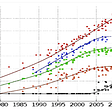## Convolutional Neural Networks and Backpropagation using Numpy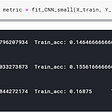## MALICIOUS URL DETECTION WITH MACHINE LEARNING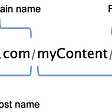## Train A XLM Roberta model for Text Classification on Pytorch## How to Land LunarLander-v2 with Proximal Policy Optimization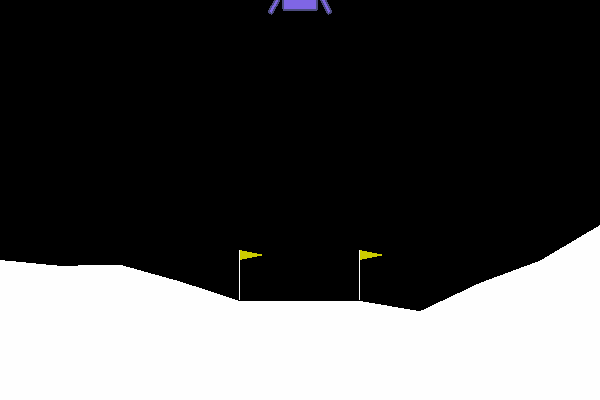## CharityML Project Solution Walkthrough —  Udacity Machine Learning Nanodegree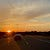## Ashley C

All things AI and emerging tech :)

## Service Robots Help the Intelligent Transformation of the Catering Industry in China## Neural Networks: the best open-source library for object detection & classification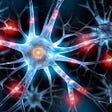## Introduction to auto encoders